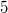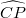﻿

### Completely positive matrices of order$5$ with a nearly$\widehat{CP}$-graph

#### Abstract

A particular class of graphs, called "nearly$\widehat{CP}$-graphs", extending the class of$\widehat{CP}$-graphs is introduced. From Barioli's characterization of completely positive matrices with a book-graph, two equivalent characterizations of completely positive matrices with a nearly$\widehat{CP}$-graph are deduced. By means of these results, new characterizations of completely positive matrices of order$5$ with a book-graph and some alternative demonstrations of known results are derived. A new characterization of completely positive matrices of order$5$ with a$\widehat{CP}$-graph and a new elementary proof of the main result obtained by Cedolin-Salce, regarding this particular class of matrices, are shown. It is also attempted to clarify some results obtained by Xu on completely positive matrices of order$5$, and it is shown by a counterexample that one of them is incorrect.

DOI Code: 10.1285/i15900932v39n1p89

Keywords: Completely positive matrices; doubly non-negative matrices; book graphs

Full Text: PDF